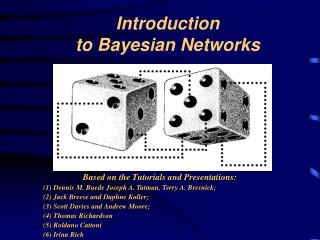# Introduction to Bayesian Networks - PowerPoint PPT PresentationDownload PresentationIntroduction to Bayesian Networks

Introduction to Bayesian NetworksDownload Presentation## Introduction to Bayesian Networks

- - - - - - - - - - - - - - - - - - - - - - - - - - - E N D - - - - - - - - - - - - - - - - - - - - - - - - - - -
##### Presentation Transcript

1. Introduction to Bayesian Networks • Based on the Tutorials and Presentations: • (1) Dennis M. Buede Joseph A. Tatman, Terry A. Bresnick; • (2) Jack Breese and Daphne Koller; • (3) Scott Davies and Andrew Moore; • (4) Thomas Richardson • (5) Roldano Cattoni • (6) Irina Rich

2. Discovering Causal Relationship from the Dynamic Environmental Data and Managing Uncertainty - are among the basic abilities of an intelligent agent Causal network with Uncertainty beliefs Dynamic Environment

3. Overview • Probabilities basic rules • Bayesian Nets • Conditional Independence • Motivating Examples • Inference in Bayesian Nets • Join Trees • Decision Making with Bayesian Networks • Learning Bayesian Networks from Data • Profiling with Bayesian Network • References and links

4. Probability of an event

5. Conditional probability

6. Conditional independence

7. The fundamental rule

8. Instance of “Fundamental rule”

9. Bayes rule

10. Bayes rule example (1) No Cancer)

11. Bayes rule example (2)

12. Overview • Probabilities basic rules • Bayesian Nets • Conditional Independence • Motivating Examples • Inference in Bayesian Nets • Join Trees • Decision Making with Bayesian Networks • Learning Bayesian Networks from Data • Profiling with Bayesian Network • References and links

13. What are Bayesian nets? • Bayesian nets (BN) are a network-based framework for representing and analyzing models involving uncertainty; • BN are different from other knowledge-based systems tools because uncertainty is handled in mathematically rigorous yet efficient and simple way • BN are different from other probabilistic analysis tools because of network representation of problems, use of Bayesian statistics, and the synergy between these

14. Definition of a Bayesian Network Knowledge structure: • variables are nodes • arcs represent probabilistic dependence between variables • conditional probabilities encode the strength of the dependencies • Computational architecture: • computes posterior probabilities given evidence about some nodes • exploits probabilistic independence for efficient computation

15. P(S) P(C|S) P(S) P(C|S)

16. cause cause • Classification: P(class|data) Text Classification Medicine symptom Bio-informatics Speech recognition symptom Computer troubleshooting Stock market What Bayesian Networks are good for? • Diagnosis: P(cause|symptom)=? • Prediction: P(symptom|cause)=? • Decision-making (given a cost function)

17. Combining domain expert knowledge with data • Incremental learning <9.7 0.6 8 14 18> <0.2 1.3 5 ?? ??> <1.3 2.8 ?? 0 1 > <?? 5.6 0 10 ??> ………………. S C • Learning causal relationships: Why learn Bayesian networks? • Efficient representation and inference • Handling missing data: <1.3 2.8 ?? 0 1 >

18. Overview • Probabilities basic rules • Bayesian Nets • Conditional Independence • Motivating Examples • Inference in Bayesian Nets • Join Trees • Decision Making with Bayesian Networks • Learning Bayesian Networks from Data • Profiling with Bayesian Network • References and links

20. Causal relationships

21. “Wet grass” example grass

22. Causal relationships grass

23. Holmes’ grass is wet ! grass E

24. Watson’s lawn is also wet ! grass E E

25. “Burglar alarm” example

26. Causal relationships

27. X1 X2 X3 X5 X6 X4 p(x1, x2, x3, x4, x5, x6) = p(x6 | x5) p(x5 | x3, x2) p(x4 | x2, x1) p(x3 | x1) p(x2 | x1) p(x1) Sample of General Product Rule

28. X1 X1 X2 X2 X3 X3 X1 X2 X1 X2 X3 X3 Arc Reversal - Bayes Rule p(x1, x2, x3) = p(x3| x1) p(x2 | x1) p(x1) p(x1, x2, x3) = p(x3 | x2, x1) p(x2) p( x1) is equivalent to is equivalent to p(x1, x2, x3) = p(x3, x2 | x1) p( x1) = p(x2 | x3, x1) p(x3 | x1) p( x1) p(x1, x2, x3) = p(x3 | x1) p(x2 , x1) = p(x3 | x1) p(x1 | x2) p( x2)

29. D-Separation of variables • Fortunately, there is a relatively simple algorithm for determining whether two variables in a Bayesian network are conditionally independent: d-separation. • Definition: X and Z are d-separated by a set of evidence variables E iff every undirected path from X to Z is “blocked”. • A path is “blocked” iff one or more of the following conditions is true: ...

30. V A path is blocked when: • There exists a variable V on the path such that • it is in the evidence set E • the arcs putting V in the path are “tail-to-tail” • Or, there exists a variable V on the path such that • it is in the evidence set E • the arcs putting V in the path are “tail-to-head” • Or, ... V

31. … a path is blocked when: • … Or, there exists a variable V on the path such that • it is NOT in the evidence set E • neither are any of its descendants • the arcs putting V on the path are “head-to-head” V

32. D-Separation and independence • Theorem [Verma & Pearl, 1998]: • If a set of evidence variables E d-separates X and Z in a Bayesian network’s graph, then X and Z will be independent. • d-separation can be computed in linear time. • Thus we now have a fast algorithm for automatically inferring whether learning the value of one variable might give us any additional hints about some other variable, given what we already know.

33. Holmes and Watson: “Wet grass” example grass E grass